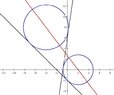# [ASK] Equation of a Circle

• MHB
• Monoxdifly

#### Monoxdifly

MHB
find standard eqations of circles that have centers on 4x+3y=8 and are tangent to both the line x+y=-2 and 7x-y=-6

What I got is $$\displaystyle 4a=–4\pm3r\sqrt2$$ and $$\displaystyle b=4\pm r\sqrt2$$. Dunno how to continue from here.

find standard eqations of circles that have centers on 4x+3y=8 and are tangent to both the line x+y=-2 and 7x-y=-6

What I got is $$\displaystyle 4a=–4\pm3r\sqrt2$$ and $$\displaystyle b=4\pm r\sqrt2$$. Dunno how to continue from here.
I'm afraid this makes no sense because there are no "a" or "b" in the original question so we have no idea how this relates to the question.

Here's what I would do:
Let (a, b) be the center of such a circle. (I think that's what you intended but did not say.) Then, since the center lies on the line 4x+ 3y= 8, we have b= (8- 4a)/3. The circle can be written $$(x- a)^2+ (y- (8-4a)/3)^2= r^2$$ and we need to find values for a and r. We have two more conditions.

If that circle crossed the line x+ y= 2 then the equation $$(x- a)^2+ (2- x- (8- 4a/3)^2= r^2$$ would have 2 solutions. But if the line is tangent to the circle, that quadratic equation must have a double root. Do the indicated squares in
$$(x- a)^2+ (2- x- (8- 4a/3)^2= r^2$$ to get the quadratic equation in standard form and use the quadratic formula to see what must be true about a and b.

Do the same with the line 7x- 4y= -6.

Wow, didn't think that the steps were far simpler than I thought, though I still needed half a day to arrive at the final answer due to my lack of accuracy during calculating some things. Thanks for your help.

...

#### Attachments

•circles_tangents.jpg
19.7 KB · Views: 30
I freaked out yesterday when my calculation got two centers of circle. Now I'm relieved that it does have two circles.